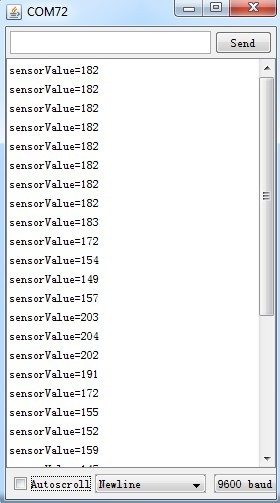••••## Galvanic Skin Response Grove Module

Description:

There are several graphs which are created in excel using GSR data.You can open the File:GSR sensor data.xls to see the detail data.Copy and paste the code below to a new Arduino sketch and upload it to Arduino.

Wear the finger sheath and relaxNow open serial monitorwe can see:Then take a deep breath. The buzzer should buzz now. And an obvious change in the output value should be observed.

The below is a graphs which is created in Excel using the data above. X axis represents time. and Y axis GSR data.

Features:

• Input Voltage: 5V/3.3V
• Sensitivity adjustable via a potentiometer
• External measuring finger cots

Mikroelectron Code:

`const int BUZZER = 3;const int GSR = A2;int threshold = 0;int sensorValue;void setup(){ long sum = 0;  Serial.begin(9600);  pinMode(BUZZEROUTPUT);  digitalWrite(BUZZERLOW);  delay(1000);  for (int i = 0; i < 500; i++)  {    sensorValue = analogRead(GSR);    sum += sensorValue;    delay(5);  }  threshold = sum / 500;  Serial.print("threshold =");  Serial.println(threshold);}void loop(){ int temp;  sensorValue = analogRead(GSR);  Serial.print("sensorValue=");  Serial.println(sensorValue);  temp = threshold - sensorValue;  if (abs(temp) > 50)  {    sensorValue = analogRead(GSR);    temp = threshold - sensorValue;    if (abs(temp) > 50)    {      digitalWrite(BUZZERHIGH);      Serial.println("YES!");      delay(3000);      digitalWrite(BUZZERLOW);      delay(1000);    }  }}`

25 JD
Quantity
1 in stock
25 JD 1+ units
23.75 JD 10+ units
22.5 JD 25+ units
21.25 JD 100+ units

## subscribe to our weekly newsletter